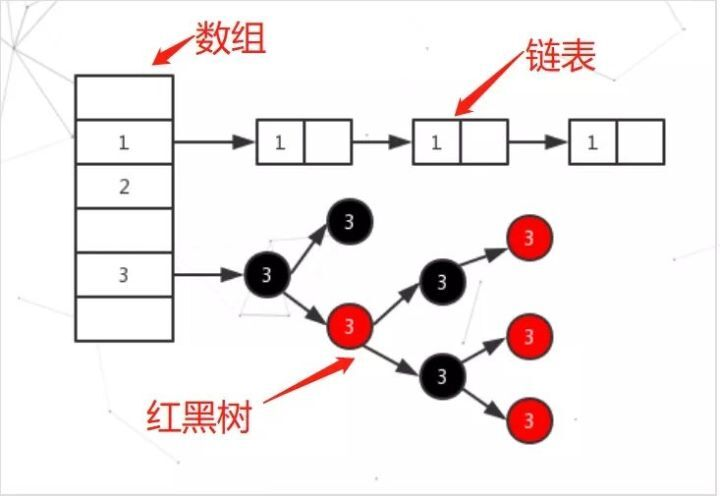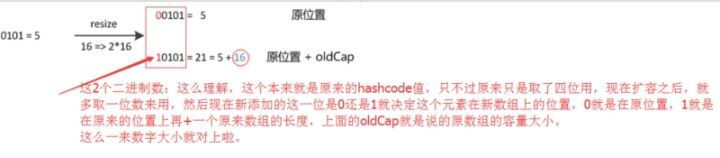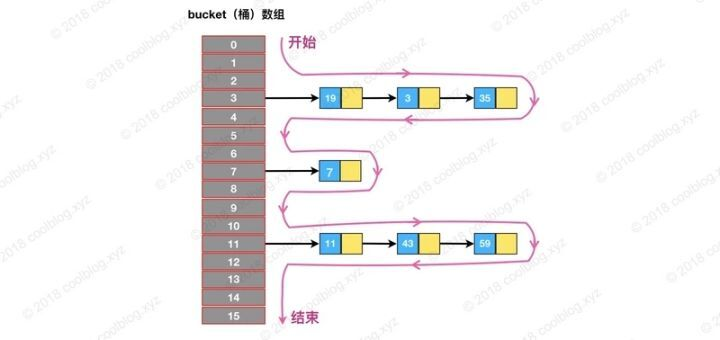# 从基础到实践，一文带你看懂 HashMap﻿

HashMap是面试中经常问到的一个知识点，也是判断一个候选人基础是否扎实的标准之一，因为通过HashMap可以引出很多知识点，比如数据结构(数组、链表、红黑树)、equals和hashcode方法，除此之外还可以引出线程安全的问题，HashMap是我在初学阶段学到的设计的最为巧妙的集合，里面有很多细节以及优化技巧都值得我们深入学习，本文将会涉及到以下问题

﻿

• 默认大小、负载因子以及扩容倍数

• 底层数据结构

• 如何处理hash冲突

• 如何计算key的hash值

• 数组长度为什么是2的幂次方

• 查找、插入、扩容过程

• fail-fast机制

﻿

﻿

﻿

## 数据结构

﻿

﻿

﻿﻿

﻿

//默认初始容量为16static final int DEFAULT_INITIAL_CAPACITY = 1 << 4; //默认负载因子为0.75static final float DEFAULT_LOAD_FACTOR = 0.75f;//Hash数组(在resize()中初始化)transient Node<K,V>[] table;//元素个数transient int size;//容量阈值(元素个数大于等于该值时会自动扩容)  int threshold;

﻿

table数组里面存放的是Node对象，Node是HashMap的一个内部类，用来表示一个key-value，源码如下：

﻿

static class Node<K,V> implements Map.Entry<K,V> { final int hash; final K key;    V value;    Node<K,V> next;﻿    Node(int hash, K key, V value, Node<K,V> next) { this.hash = hash; this.key = key; this.value = value; this.next = next;    }  public final K getKey()        { return key; } public final V getValue()      { return value; } public final String toString() { return key + "=" + value; } public final int hashCode() { return Objects.hashCode(key) ^ Objects.hashCode(value);//^表示相同返回0，不同返回1 //Objects.hashCode(o)————>return o != null ? o.hashCode() : 0;    }﻿ public final V setValue(V newValue) {        V oldValue = value;        value = newValue; return oldValue;    }﻿ public final boolean equals(Object o) { if (o == this) return true; if (o instanceof Map.Entry) {            Map.Entry<?,?> e = (Map.Entry<?,?>)o; //Objects.equals(1,b)————> return (a == b) || (a != null && a.equals(b)); if (Objects.equals(key, e.getKey()) && Objects.equals(value, e.getValue())) return true;        } return false;    }}

﻿

﻿

• 默认初始容量为16，默认负载因子为0.75

• threshold = 数组长度 * loadFactor，当元素个数大于等于threshold(容量阈值)时，HashMap会进行扩容操作

• table数组中存放指向链表的引用

﻿

﻿

﻿

﻿

## table数组长度永远为2的幂次方

﻿

﻿

﻿

/*找到大于或等于 cap 的最小2的幂，用来做容量阈值*/static final int tableSizeFor(int cap) {    int n = cap - 1;    n |= n >>> 1;    n |= n >>> 2;    n |= n >>> 4;    n |= n >>> 8;    n |= n >>> 16;    return (n < 0) ? 1 : (n >= MAXIMUM_CAPACITY) ? MAXIMUM_CAPACITY : n + 1;}

﻿

tableSizeFor的功能（不考虑大于最大容量的情况）是返回大于等于输入参数且最近的2的整数次幂的数。比如10，则返回16。

﻿

﻿

﻿

﻿

﻿

﻿

﻿

/*传入初始容量和负载因子*/public HashMap(int initialCapacity, float loadFactor) {  if (initialCapacity < 0) throw new IllegalArgumentException("Illegal initial capacity: " +initialCapacity); if (initialCapacity > MAXIMUM_CAPACITY)        initialCapacity = MAXIMUM_CAPACITY; if (loadFactor <= 0 || Float.isNaN(loadFactor)) throw new IllegalArgumentException("Illegal load factor: " +loadFactor);  this.loadFactor = loadFactor; this.threshold = tableSizeFor(initialCapacity);}

﻿

### 那么为什么要把数组长度设计为2的幂次方呢？

﻿

﻿

1、当数组长度为2的幂次方时，可以使用位运算来计算元素在数组中的下标

﻿

HashMap是通过index=hash&(table.length-1)这条公式来计算元素在table数组中存放的下标，就是把元素的hash值和数组长度减1的值做一个与运算，即可求出该元素在数组中的下标，这条公式其实等价于hash%length，也就是对数组长度求模取余，只不过只有当数组长度为2的幂次方时，hash&(length-1)才等价于hash%length，使用位运算可以提高效率。

﻿

2、 增加hash值的随机性，减少hash冲突

﻿

﻿

## 扩容

﻿

HashMap每次扩容都是建立一个新的table数组，长度和容量阈值都变为原来的两倍，然后把原数组元素重新映射到新数组上，具体步骤如下：

﻿

1. 首先会判断table数组长度，如果大于0说明已被初始化过，那么按当前table数组长度的2倍进行扩容，阈值也变为原来的2倍

2. 若table数组未被初始化过，且threshold(阈值)大于0说明调用了HashMap(initialCapacity, loadFactor)构造方法，那么就把数组大小设为threshold

3. 若table数组未被初始化，且threshold为0说明调用HashMap()构造方法，那么就把数组大小设为16，threshold设为16*0.75

4. 接着需要判断如果不是第一次初始化，那么扩容之后，要重新计算键值对的位置，并把它们移动到合适的位置上去，如果节点是红黑树类型的话则需要进行红黑树的拆分。

﻿

﻿

﻿﻿

﻿

/*扩容*/final Node<K,V>[] resize() {    Node<K,V>[] oldTab = table; int oldCap = (oldTab == null) ? 0 : oldTab.length; int oldThr = threshold; int newCap, newThr = 0;  //1、若oldCap>0 说明hash数组table已被初始化 if (oldCap > 0) { if (oldCap >= MAXIMUM_CAPACITY) {            threshold = Integer.MAX_VALUE; return oldTab;        }//按当前table数组长度的2倍进行扩容，阈值也变为原来的2倍 else if ((newCap = oldCap << 1) < MAXIMUM_CAPACITY && oldCap >= DEFAULT_INITIAL_CAPACITY)            newThr = oldThr << 1;     }//2、若数组未被初始化，而threshold>0说明调用了HashMap(initialCapacity)和HashMap(initialCapacity, loadFactor)构造器 else if (oldThr > 0)        newCap = oldThr;//新容量设为数组阈值 else { //3、若table数组未被初始化，且threshold为0说明调用HashMap()构造方法                     newCap = DEFAULT_INITIAL_CAPACITY;//默认为16        newThr = (int)(DEFAULT_LOAD_FACTOR * DEFAULT_INITIAL_CAPACITY);//16*0.75    }  //若计算过程中，阈值溢出归零，则按阈值公式重新计算 if (newThr == 0) { float ft = (float)newCap * loadFactor;        newThr = (newCap < MAXIMUM_CAPACITY && ft < (float)MAXIMUM_CAPACITY ?                  (int)ft : Integer.MAX_VALUE);    }    threshold = newThr; //创建新的hash数组，hash数组的初始化也是在这里完成的    Node<K,V>[] newTab = (Node<K,V>[])new Node[newCap];    table = newTab; //如果旧的hash数组不为空，则遍历旧数组并映射到新的hash数组 if (oldTab != null) { for (int j = 0; j < oldCap; ++j) {            Node<K,V> e; if ((e = oldTab[j]) != null) {                oldTab[j] = null;//GC if (e.next == null)//如果只链接一个节点，重新计算并放入新数组                    newTab[e.hash & (newCap - 1)] = e; //若是红黑树，则需要进行拆分     else if (e instanceof TreeNode)                    ((TreeNode<K,V>)e).split(this, newTab, j, oldCap); else {  //rehash————>重新映射到新数组                    Node<K,V> loHead = null, loTail = null;                    Node<K,V> hiHead = null, hiTail = null;                    Node<K,V> next; do {                        next = e.next; /*注意这里使用的是：e.hash & oldCap，若为0则索引位置不变，不为0则新索引=原索引+旧数组长度*/ if ((e.hash & oldCap) == 0) { if (loTail == null)                                loHead = e; else                                loTail.next = e;                            loTail = e;                        } else { if (hiTail == null)                                hiHead = e; else                                hiTail.next = e;                            hiTail = e;                        }                    } while ((e = next) != null); if (loTail != null) {                        loTail.next = null;                        newTab[j] = loHead;                    } if (hiTail != null) {                        hiTail.next = null;                        newTab[j + oldCap] = hiHead;                    }                }            }        }    } return newTab;}

﻿

﻿

### 链表树化

﻿

﻿

• 链表长度大于等于8

• table数组长度大于等于64

﻿

﻿

﻿

﻿

﻿

﻿

## 查找

﻿

﻿

1. 首先通过自定义的hash方法计算出key的hash值，求出在数组中的位置

2. 判断该位置上是否有节点，若没有则返回null，代表查询不到指定的元素

3. 若有则判断该节点是不是要查找的元素，若是则返回该节点

4. 若不是则判断节点的类型，如果是红黑树的话，则调用红黑树的方法去查找元素

5. 如果是链表类型，则遍历链表调用equals方法去查找元素

﻿

HashMap的查找是非常快的，要查找一个元素首先得知道key的hash值，在HashMap中并不是直接通过key的hashcode方法获取哈希值，而是通过内部自定义的hash方法计算哈希值，我们来看看其实现：

﻿

static final int hash(Object key) {    int h; return (key == null) ? 0 : (h = key.hashCode()) ^ (h >>> 16);}

﻿

(h = key.hashCode()) ^ (h >>> 16) 是为了让高位数据与低位数据进行异或，变相的让高位数据参与到计算中，int有 32 位，右移16位就能让低16位和高16位进行异或，也是为了增加hash值的随机性。

﻿

﻿

public V get(Object key) {    Node<K,V> e; return (e = getNode(hash(key), key)) == null ? null : e.value;//hash(key)不等于key.hashCode}﻿final Node<K,V> getNode(int hash, Object key) {    Node<K,V>[] tab; //指向hash数组    Node<K,V> first, e; //first指向hash数组链接的第一个节点，e指向下一个节点    int n;//hash数组长度    K k; /*(n - 1) & hash ————>根据hash值计算出在数组中的索引index（相当于对数组长度取模，这里用位运算进行了优化）*/ if ((tab = table) != null && (n = tab.length) > 0 && (first = tab[(n - 1) & hash]) != null) { //基本类型用==比较，其它用equals比较 if (first.hash == hash && ((k = first.key) == key || (key != null && key.equals(k)))) return first; if ((e = first.next) != null) { //如果first是TreeNode类型，则调用红黑树查找方法 if (first instanceof TreeNode) return ((TreeNode<K,V>)first).getTreeNode(hash, key); do {//向后遍历 if (e.hash == hash && ((k = e.key) == key || (key != null && key.equals(k)))) return e;            } while ((e = e.next) != null);        }    } return null;}

﻿

﻿

## 插入

﻿

﻿

1. 当table数组为空时，通过扩容的方式初始化table

2. 通过计算键的hash值求出下标后，若该位置上没有元素(没有发生hash冲突)，则新建Node节点插入

3. 若发生了hash冲突，遍历链表查找要插入的key是否已经存在，存在的话根据条件判断是否用新值替换旧值

4. 如果不存在，则将元素插入链表尾部，并根据链表长度决定是否将链表转为红黑树

5. 判断键值对数量是否大于等于阈值，如果是的话则进行扩容操作

﻿

﻿

public V put(K key, V value) { return putVal(hash(key), key, value, false, true);}﻿final V putVal(int hash, K key, V value, boolean onlyIfAbsent,boolean evict) {    Node<K,V>[] tab;//指向hash数组    Node<K,V> p;//初始化为table中第一个节点 int n, i;//n为数组长度，i为索引  //tab被延迟到插入新数据时再进行初始化 if ((tab = table) == null || (n = tab.length) == 0)        n = (tab = resize()).length; //如果数组中不包含Node引用，则新建Node节点存入数组中即可     if ((p = tab[i = (n - 1) & hash]) == null)        tab[i] = newNode(hash, key, value, null);//new Node<>(hash, key, value, next) else {        Node<K,V> e; //如果要插入的key-value已存在，用e指向该节点        K k; //如果第一个节点就是要插入的key-value，则让e指向第一个节点（p在这里指向第一个节点） if (p.hash == hash && ((k = p.key) == key || (key != null && key.equals(k))))            e = p; //如果p是TreeNode类型，则调用红黑树的插入操作（注意：TreeNode是Node的子类） else if (p instanceof TreeNode)            e = ((TreeNode<K,V>)p).putTreeVal(this, tab, hash, key, value); else { //对链表进行遍历，并用binCount统计链表长度 for (int binCount = 0; ; ++binCount) { //如果链表中不包含要插入的key-value，则将其插入到链表尾部 if ((e = p.next) == null) {                    p.next = newNode(hash, key, value, null); //如果链表长度大于或等于树化阈值，则进行树化操作 if (binCount >= TREEIFY_THRESHOLD - 1)                        treeifyBin(tab, hash); break;                } //如果要插入的key-value已存在则终止遍历，否则向后遍历 if (e.hash == hash && ((k = e.key) == key || (key != null && key.equals(k)))) break;                p = e;            }        } //如果e不为null说明要插入的key-value已存在 if (e != null) {            V oldValue = e.value; //根据传入的onlyIfAbsent判断是否要更新旧值 if (!onlyIfAbsent || oldValue == null)                e.value = value;            afterNodeAccess(e); return oldValue;        }    }    ++modCount; //键值对数量大于等于阈值时，则进行扩容 if (++size > threshold)        resize();    afterNodeInsertion(evict);//也是空函数？回调？不知道干嘛的 return null;}

﻿

﻿

﻿

## 删除

﻿

HashMap的删除操作并不复杂，仅需三个步骤即可完成。

﻿

1. 定位桶位置

2. 遍历链表找到相等的节点

3. 第三步删除节点

﻿

public V remove(Object key) {    Node<K,V> e; return (e = removeNode(hash(key), key, null, false, true)) == null ? null : e.value;}﻿final Node<K,V> removeNode(int hash, Object key, Object value,boolean matchValue, boolean movable) {    Node<K,V>[] tab;     Node<K,V> p;  int n, index; //1、定位元素桶位置 if ((tab = table) != null && (n = tab.length) > 0 && (p = tab[index = (n - 1) & hash]) != null) {        Node<K,V> node = null, e;         K k;         V v; // 如果键的值与链表第一个节点相等，则将 node 指向该节点 if (p.hash == hash && ((k = p.key) == key || (key != null && key.equals(k))))            node = p; else if ((e = p.next) != null) {   // 如果是 TreeNode 类型，调用红黑树的查找逻辑定位待删除节点 if (p instanceof TreeNode)                node = ((TreeNode<K,V>)p).getTreeNode(hash, key); else { // 2、遍历链表，找到待删除节点 do { if (e.hash == hash && ((k = e.key) == key || (key != null && key.equals(k)))) {                        node = e; break;                    }                    p = e;                } while ((e = e.next) != null);            }        }         // 3、删除节点，并修复链表或红黑树 if (node != null && (!matchValue || (v = node.value) == value || (value != null && value.equals(v)))) { if (node instanceof TreeNode)                ((TreeNode<K,V>)node).removeTreeNode(this, tab, movable); else if (node == p)                tab[index] = node.next; else                p.next = node.next;            ++modCount;            --size;            afterNodeRemoval(node); return node;        }    } return null;}

﻿

﻿

## 遍历

﻿

﻿

﻿

    Map<String, Integer> map = new HashMap<>(); map.put("1", 1); map.put("2", 2); map.put("3", 3); for (String s : map.keySet()) { if (s.equals("2"))  map.remove("2");        }

﻿

﻿

transient int modCount;

﻿

﻿

﻿

public Set<K> keySet() {    Set<K> ks = keySet; if (ks == null) {        ks = new KeySet();        keySet = ks;    } return ks;}﻿final class KeySet extends AbstractSet<K> {   public final Iterator<K> iterator()     { return new KeyIterator(); }  // 省略部分代码}﻿final class KeyIterator extends HashIterator implements Iterator<K> { public final K next() { return nextNode().key; }}﻿/*HashMap迭代器基类，子类有KeyIterator、ValueIterator等*/abstract class HashIterator {    Node<K,V> next;        //下一个节点    Node<K,V> current;     //当前节点 int expectedModCount;  //修改次数 int index;             //当前索引 //无参构造    HashIterator() {        expectedModCount = modCount;        Node<K,V>[] t = table;        current = next = null;        index = 0; //找到第一个不为空的桶的索引 if (t != null && size > 0) { do {} while (index < t.length && (next = t[index++]) == null);        }    } //是否有下一个节点 public final boolean hasNext() { return next != null;    } //返回下一个节点 final Node<K,V> nextNode() {        Node<K,V>[] t;        Node<K,V> e = next; if (modCount != expectedModCount) throw new ConcurrentModificationException();//fail-fast if (e == null) throw new NoSuchElementException(); //当前的链表遍历完了就开始遍历下一个链表 if ((next = (current = e).next) == null && (t = table) != null) { do {} while (index < t.length && (next = t[index++]) == null);        } return e;    } //删除元素 public final void remove() {        Node<K,V> p = current; if (p == null) throw new IllegalStateException(); if (modCount != expectedModCount) throw new ConcurrentModificationException();        current = null;        K key = p.key;        removeNode(hash(key), key, null, false, false);//调用外部的removeNode        expectedModCount = modCount;    }}

﻿

﻿

if (modCount != expectedModCount) throw new ConcurrentModificationException();

﻿

﻿

﻿

removeNode(hash(key), key, null, false, false);//调用外部的removeNodeexpectedModCount = modCount;

﻿

﻿

Map<String, Integer> map = new HashMap<>();        map.put("1", 1);        map.put("2", 2);        map.put("3", 3);        Iterator<String> iterator = map.keySet().iterator(); while (iterator.hasNext()){ if (iterator.next().equals("2"))                iterator.remove();        }

﻿

﻿

﻿﻿

## equasl和hashcode

﻿

﻿

﻿

﻿

public class Person {    Integer id;    String name;  public Person(Integer id, String name) { this.id = id; this.name = name;    }﻿ @Override public boolean equals(Object obj) { if (obj == null) return false; if (obj == this) return true; if (obj instanceof Person) {            Person person = (Person) obj; if (this.id == person.id) return true;        } return false;    }﻿ public static void main(String[] args) {        Person p1 = new Person(1, "aaa");        Person p2 = new Person(1, "bbb");        HashMap<Person, String> map = new HashMap<>();        map.put(p1, "这是p1");        System.out.println(map.get(p2));    }}

﻿

• 原生的equals方法是使用==来比较对象的

• 原生的hashCode值是根据内存地址换算出来的一个值

﻿

Person类重写equals方法来根据id判断是否相等，当没有重写hashcode方法时，插入p1后便无法用p2取出元素，这是因为p1和p2的哈希值不相等。

﻿

HashMap插入元素时是根据元素的哈希值来确定存放在数组中的位置，因此HashMap的key需要重写equals和hashcode方法。

﻿

## 总结

﻿

﻿

﻿

﻿## 评论